## DataFrame(13)：DataFrame的排序与排名问题_dataframe 排序_数据分析与统计学之美的博客-程序员秘密

#### 1、说明

DataFrame中的排序分为两种，一种是对索引排序，一种是对值进行排序。
索引排序：sort_index()；值排序：sort_values()；值排名：rank()
对于索引排序，涉及到对行索引、列索引的排序，并且还涉及到是升序还是降序。函数df.sort_index(axis= , ascending= , inplace=)，需要特别注意这三个参数。axis表示对行操作，还是对列操作；ascending表示升序，还是降序操作。
对于值排序，同样也是涉及到行、列排序问题，升序、降序排列问题。函数df.sort_values(by= , axis= , ascending= , inplace=),也需要特别注意这几个参数，只是多了一个by操作，需要我们指明是按照哪一行或哪一列，进行排序的。
注意：axis=0表示对行操作，axis=1表示对列进行操作；ascending=True表示升序，ascending=False表示降序；inplace=True表示对原始DataFrame本身操作，因此不需要赋值操作，inplace=False相当于是对原始DataFrame的拷贝，之后的一些操作都是针对这个拷贝文件进行操作的，因此需要我们赋值给一个变量，保存操作后的结果。

#### 2、索引排序：df.sort_index()

##### ① 对行索引，进行升序排列
``````df = pd.DataFrame({
"A":[1,3,5,7,9],
"D":[1,2,3,4,5],
"C":[3,6,9,12,15],
"B":[2,4,6,8,10]},
index=list("acbed"))
display(df)
display(id(df))

df.sort_index(axis=0,ascending=True,inplace=True)
display(df)
display(id(df))

df1 = df.sort_index(axis=0,ascending=True)
display(df1)
display(id(df1))
``````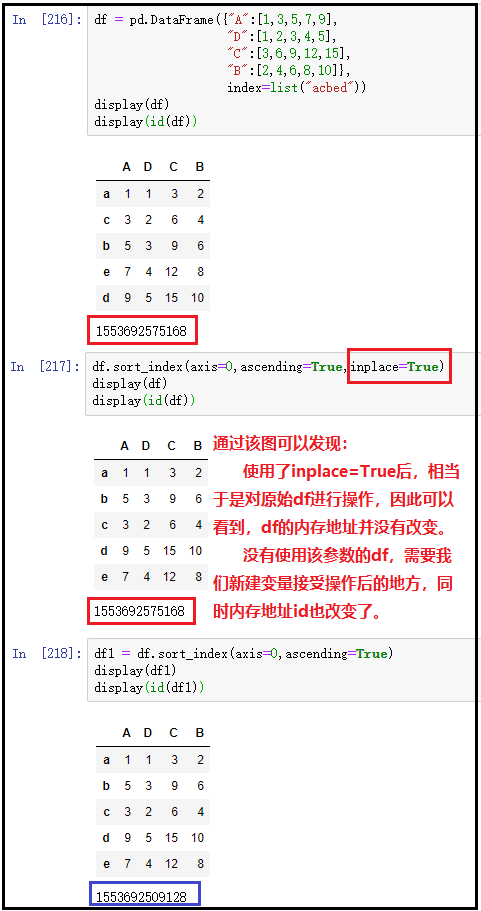##### ② 对列索引，进行降序排列
``````df = pd.DataFrame({
"A":[1,3,5,7,9],
"D":[1,2,3,4,5],
"C":[3,6,9,12,15],
"B":[2,4,6,8,10]},
index=list("acbed"))
display(df)

df.sort_index(axis=1,ascending=False,inplace=True)
display(df)
``````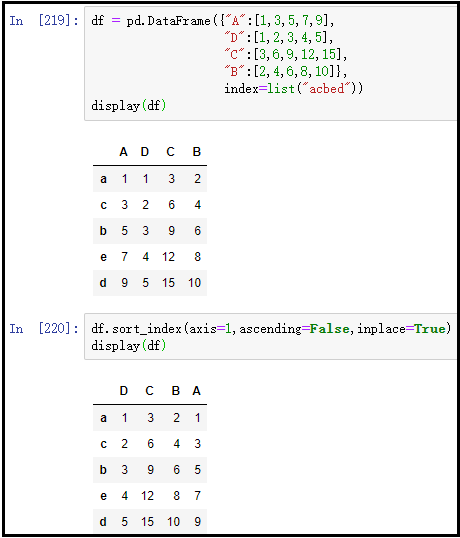#### 3、值排序：df.sort_values()

##### ① 对某一列进行升序排列(有实际意义)
``````df = pd.DataFrame({
"A":[3,1,5,9,7],
"D":[4,1,2,5,3],
"C":[3,15,9,6,12],
"B":[2,4,6,10,8]},
index=list("acbed"))
display(df)

df.sort_values(by="A",axis=0,ascending=True,inplace=True)
display(df)
``````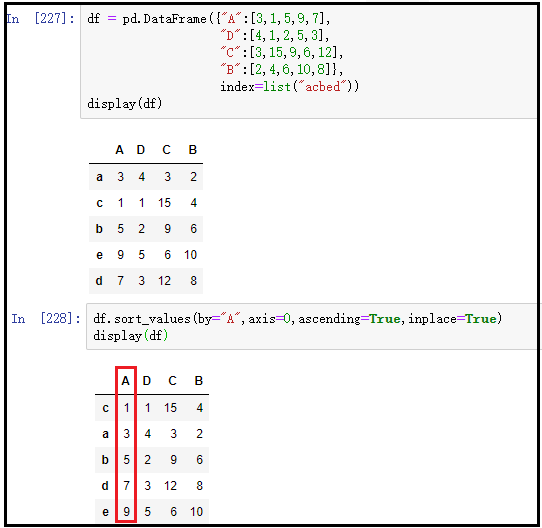##### ② 对某一行进行降序排列(实际意义不大)
``````df = pd.DataFrame({
"A":[3,1,5,9,7],
"D":[4,1,2,5,3],
"C":[3,15,9,6,12],
"B":[2,4,6,10,8]},
index=list("acbed"))
display(df)

df.sort_values(by="A",axis=1,ascending=False,inplace=True)
display(df)
``````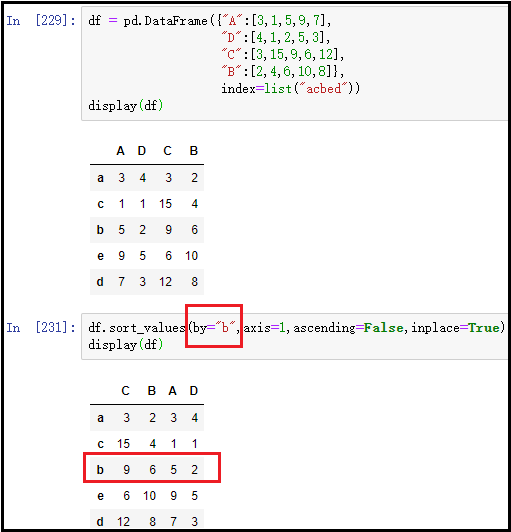##### ③ 对多列进行联合排序(重要)
``````df = pd.DataFrame({
"A":[3,1,3,9,7],
"D":[666,1,888,5,3],
"C":[3,15,9,6,12],
"B":[2,4,6,10,8]},
index=list("acbed"))
display(df)

df.sort_values(by=["A","D"],axis=0,ascending=[True,False],inplace=True)
df
``````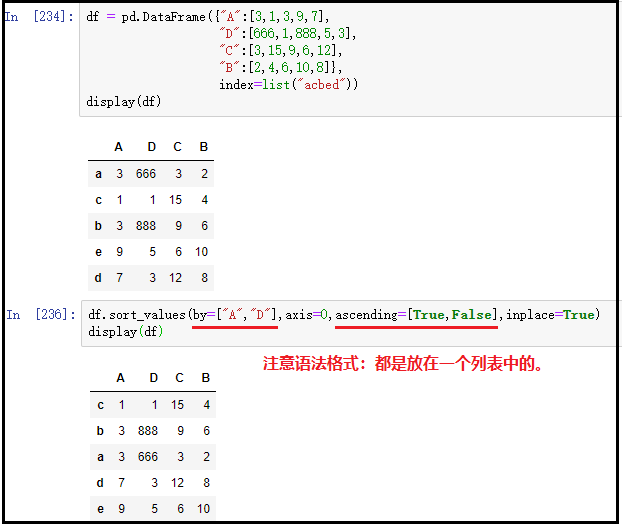#### 4、sort_values()中的na_position参数

na_position参数用于设定缺失值的显示位置，first表示缺失值显示在最前面；last表示缺失值显示在最后面。

``````df = pd.DataFrame({
"A":[10,8,np.nan,2,4],
"D":[1,7,5,3,8],
"B":[5,2,8,4,1]},
index=list("abcde"))
display(df)

df.sort_values(by="A",axis=0,inplace=True,na_position="first")
display(df)

df.sort_values(by="A",axis=0,inplace=True,na_position="last")
display(df)
``````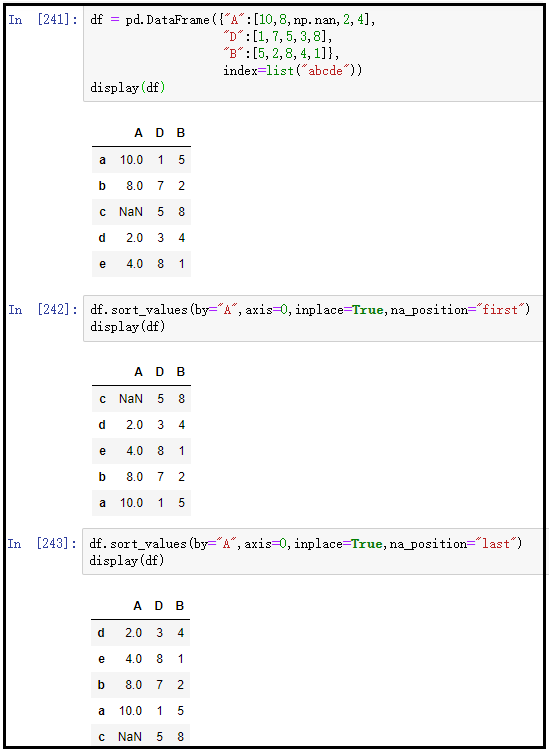#### 5、“值排名”：rank()函数

##### 1）rank()函数的常用参数说明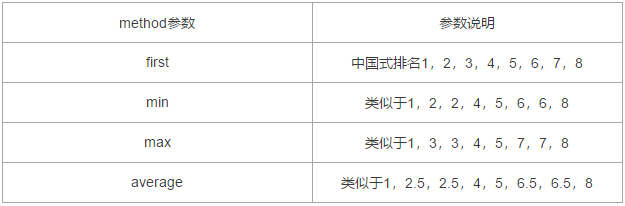##### 2）原始数据
``````x = {
"name":["张三","李四","王五","赵六","郑七","陈八","黄九","孙十"],
"sales":[60,40,50,40,30,80,70,60]}
df = pd.DataFrame(x)
display(df)
``````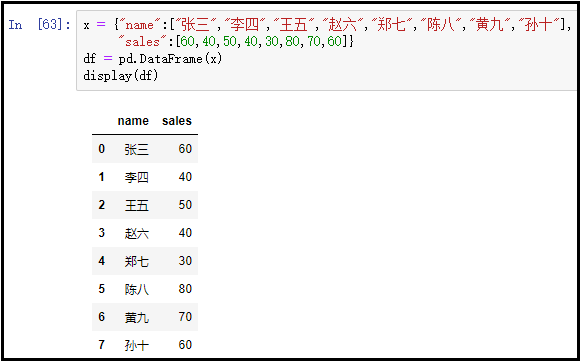##### 3）rank()函数使用如下
###### ① method=“first”
``````x = {
"name":["张三","李四","王五","赵六","郑七","陈八","黄九","孙十"],
"sales":[60,40,50,40,30,80,70,60]}
df = pd.DataFrame(x)
display(df)

df["排名"] = df["sales"].rank(method="first")
display(df)
``````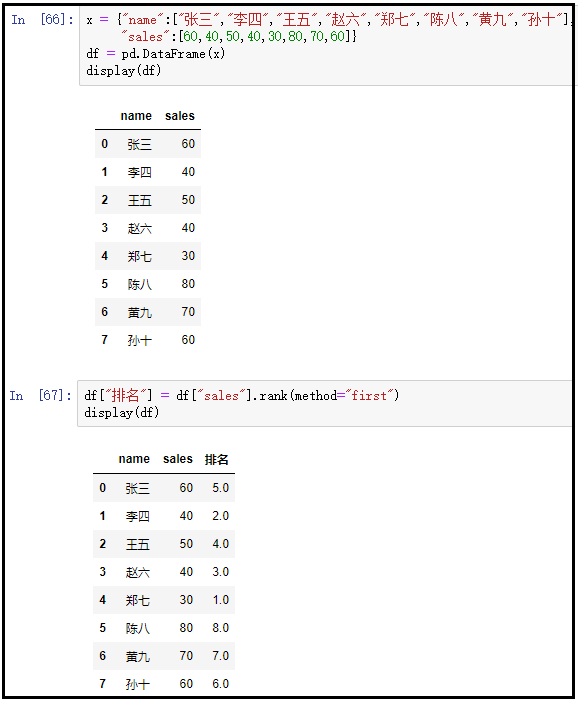###### ② method=“min”
``````x = {
"name":["张三","李四","王五","赵六","郑七","陈八","黄九","孙十"],
"sales":[60,40,50,40,30,80,70,60]}
df = pd.DataFrame(x)
display(df)

df["排名"] = df["sales"].rank(method="min")
display(df)
``````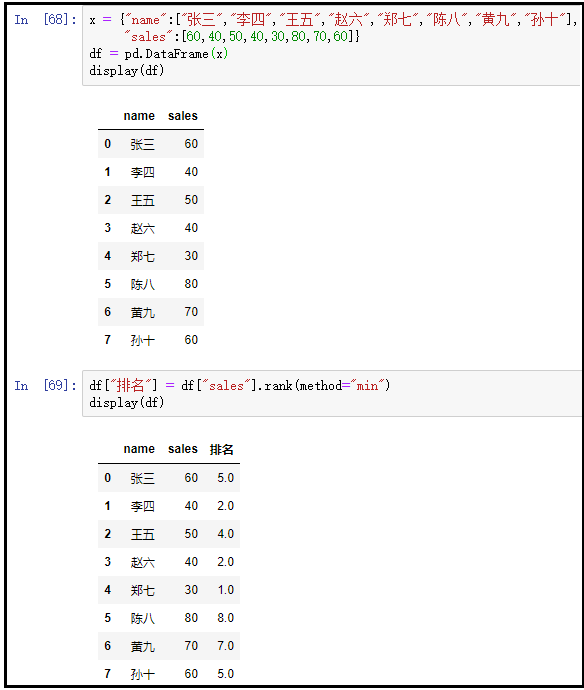###### ③ method=“max”
``````x = {
"name":["张三","李四","王五","赵六","郑七","陈八","黄九","孙十"],
"sales":[60,40,50,40,30,80,70,60]}
df = pd.DataFrame(x)
display(df)

df["排名"] = df["sales"].rank(method="max")
display(df)
``````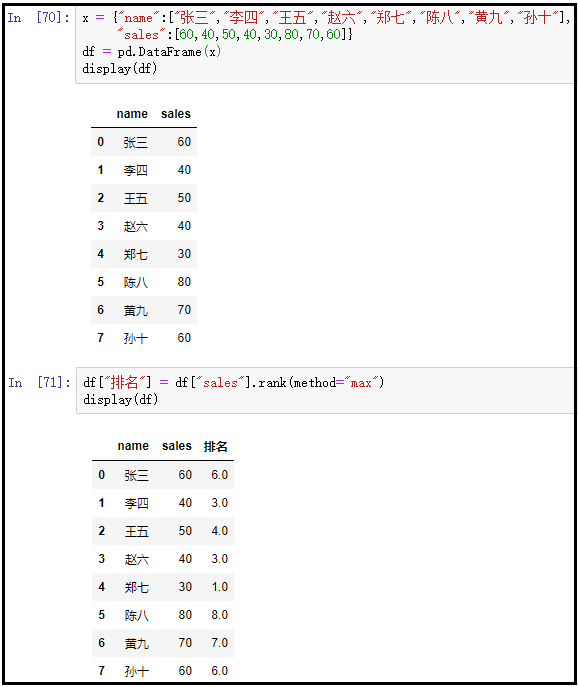###### ④ method=“average”
``````x = {
"name":["张三","李四","王五","赵六","郑七","陈八","黄九","孙十"],
"sales":[60,40,50,40,30,80,70,60]}
df = pd.DataFrame(x)
display(df)

df["排名"] = df["sales"].rank(method="average")
display(df)
``````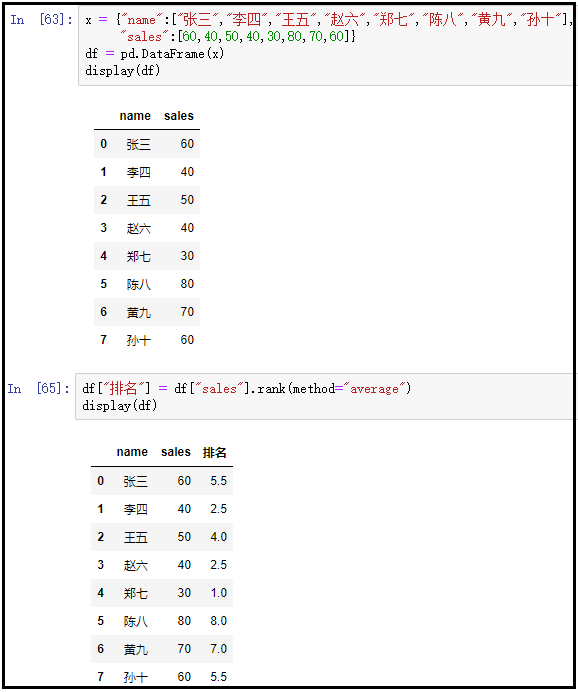rgb转16进制

### linux服务器操作系统推荐 CentOS vs Ubuntu_ubuntu centos 发展_csdnfan的博客-程序员秘密

Ubuntu和CentOS之间选哪个是老生常谈了，在知乎上也有好多争论。我在这里只列出一些区别并不做谁更好的结论，只有更适合的情况。1、Ubuntu是基于Debian架构的，而CentOS是从Red Hat Enterprise Linux派生的。这一点决定了两个系统在多方面必然不同，例如安装软件上Ubuntu使用apt-get软件包管理器下载DEB软件包，CentOS使用yum命令从中央存储库下载并安装RPM软件包。2、Ubuntu追求的是新技术，CentOS则比较老成求稳。Ubuntu上的软

### mybatis常用的集中判断语句_mybaitis里面判断语句_qq_42556903的博客-程序员秘密

&nbsp;属性是存在&nbsp;属性不存在&nbsp;属性值是null&nbsp;判断Collection.size&lt;1或String.length()&lt;1&nbsp;等于&nbsp;不等于&nbsp;大于&nbsp;大于等于&nbsp;小于&nbsp;小于等于、&nbsp;&nbsp;&nbsp;&nbsp; select id,note from Prod...

### CTF必备技能丨Linux Pwn入门教程——ROP技术（下）_dfdhxb995397的博客-程序员秘密

Linux Pwn入门教程系列分享如约而至，本套课程是作者依据i春秋Pwn入门课程中的技术分类，并结合近几年赛事中出现的题目和文章整理出一份相对完整的Linux Pwn教程。教程仅针对i386/amd64下的Linux Pwn常见的Pwn手法，如栈，堆，整数溢出，格式化字符串，条件竞争等进行介绍，所有环境都会封装在Docker镜像当中，并提供调试用的教学程序，来自历年赛事的原题和带有...

### mysql使用WITH ROLLUP分组并统计数据_with rollup统计人数_wooden_people的博客-程序员秘密

WITH ROLLUP 可以实现在分组统计数据基础上再进行相同的统计（SUM,AVG,COUNT…）。mysql&gt; SELECT * FROM employee_tbl;+----+--------+---------------------+--------+| id | name | date | singin |+----+--------...

### checkbox_weixin_45789779的博客-程序员秘密

.circle-check,.square-check { display: inline-block;}.circle-check&gt;input[type="checkbox"] { width: 1.64rem; height: 1.64rem; display: inline-block; text-align: center; v...